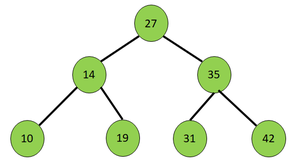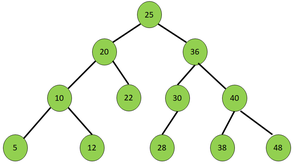Related Articles
Print nodes of a Binary Search Tree in Top Level Order and Reversed Bottom Level Order alternately
• Difficulty Level : Expert
• Last Updated : 22 Sep, 2020

Given a Binary Search Tree, the task is to print the nodes of the BST in the following order:

• If the BST contains levels numbered from 1 to N then, the printing order is level 1, level N, level 2, level N – 1, and so on.
• The top-level order (1, 2, …) nodes are printed from left to right, while the bottom level order (N, N-1, …) nodes are printed from right to left.

Examples:

Input:Output: 27 42 31 19 10 14 35
Explanation:
Level 1 from left to right: 27
Level 3 from right to left: 42 31 19 10
Level 2 from left to right: 14 35

Input:Output: 25 48 38 28 12 5 20 36 40 30 22 10

## Recommended: Please try your approach on {IDE} first, before moving on to the solution.

Approach: To solve the problem, the idea is to store the nodes of BST in ascending and descending order of levels and node values and print all the nodes of the same level alternatively between ascending and descending order. Follow the steps below to solve the problem:

• Initialize a Min Heap and a Max Heap to store the nodes in ascending and descending order of level and node values respectively.
• Perform a level order traversal on the given BST to store the nodes in the respective priority queues.
• Print all the nodes of each level one by one from the Min Heap followed by the Max Heap alternately.
• If any level in the Min Heap or Max Heap is found to be already printed, skip to the next level.

Below is the implementation of the above approach:

## C++

 `// C++ program for the above approach`` ` `#include ``using` `namespace` `std;`` ` `// Structure of a BST node``struct` `node {``    ``int` `data;``    ``struct` `node* left;``    ``struct` `node* right;``};`` ` `// Utility function to create a new BST node``struct` `node* newnode(``int` `d)``{``    ``struct` `node* temp``        ``= (``struct` `node*)``malloc``(``sizeof``(``struct` `node));``    ``temp->left = NULL;``    ``temp->right = NULL;``    ``temp->data = d;``    ``return` `temp;``}`` ` `// Function to print the nodes of a``// BST in Top Level Order and Reversed``// Bottom Level Order alternatively``void` `printBST(node* root)``{``    ``// Stores the nodes in descending order``    ``// of the level and node values``    ``priority_queue > great;`` ` `    ``// Stores the nodes in ascending order``    ``// of the level and node values`` ` `    ``priority_queue,``                   ``vector >,``                   ``greater > >``        ``small;`` ` `    ``// Initialize a stack for``    ``// level order traversal``    ``stack > st;`` ` `    ``// Push the root of BST``    ``// into the stack``    ``st.push({ root, 1 });`` ` `    ``// Perform Level Order Traversal``    ``while` `(!st.empty()) {`` ` `        ``// Extract and pop the node``        ``// from the current level``        ``node* curr = st.top().first;`` ` `        ``// Stores level of current node``        ``int` `level = st.top().second;``        ``st.pop();`` ` `        ``// Store in the priority queues``        ``great.push({ level, curr->data });``        ``small.push({ level, curr->data });`` ` `        ``// Traverse left subtree``        ``if` `(curr->left)``            ``st.push({ curr->left, level + 1 });`` ` `        ``// Traverse right subtree``        ``if` `(curr->right)``            ``st.push({ curr->right, level + 1 });``    ``}`` ` `    ``// Stores the levels that are printed``    ``unordered_set<``int``> levelsprinted;`` ` `    ``// Print the nodes in the required manner``    ``while` `(!small.empty() && !great.empty()) {`` ` `        ``// Store the top level of traversal``        ``int` `toplevel = small.top().first;`` ` `        ``// If the level is already printed``        ``if` `(levelsprinted.find(toplevel)``            ``!= levelsprinted.end())``            ``break``;`` ` `        ``// Otherwise``        ``else``            ``levelsprinted.insert(toplevel);`` ` `        ``// Print nodes of same level``        ``while` `(!small.empty()``               ``&& small.top().first == toplevel) {``            ``cout << small.top().second << ``" "``;``            ``small.pop();``        ``}`` ` `        ``// Store the bottom level of traversal``        ``int` `bottomlevel = great.top().first;`` ` `        ``// If the level is already printed``        ``if` `(levelsprinted.find(bottomlevel)``            ``!= levelsprinted.end()) {``            ``break``;``        ``}``        ``else` `{``            ``levelsprinted.insert(bottomlevel);``        ``}`` ` `        ``// Print the nodes of same level``        ``while` `(!great.empty()``               ``&& great.top().first == bottomlevel) {``            ``cout << great.top().second << ``" "``;``            ``great.pop();``        ``}``    ``}``}`` ` `// Driver Code``int` `main()``{``    ``/*``    ``Given BST`` ` `                                ``25``                              ``/     \``                            ``20      36``                           ``/  \      / \``                          ``10   22   30 40``                         ``/  \      /   / \``                        ``5   12    28  38 48``    ``*/`` ` `    ``// Creating the BST``    ``node* root = newnode(25);``    ``root->left = newnode(20);``    ``root->right = newnode(36);``    ``root->left->left = newnode(10);``    ``root->left->right = newnode(22);``    ``root->left->left->left = newnode(5);``    ``root->left->left->right = newnode(12);``    ``root->right->left = newnode(30);``    ``root->right->right = newnode(40);``    ``root->right->left->left = newnode(28);``    ``root->right->right->left = newnode(38);``    ``root->right->right->right = newnode(48);`` ` `    ``// Function Call``    ``printBST(root);`` ` `    ``return` `0;``}`
Output:
```25 48 38 28 12 5 20 36 40 30 22 10
```

Time Complexity: O(V log(V)), where V denotes the number of vertices in the given Binary Tree
Auxiliary Space: O(V)

My Personal Notes arrow_drop_up